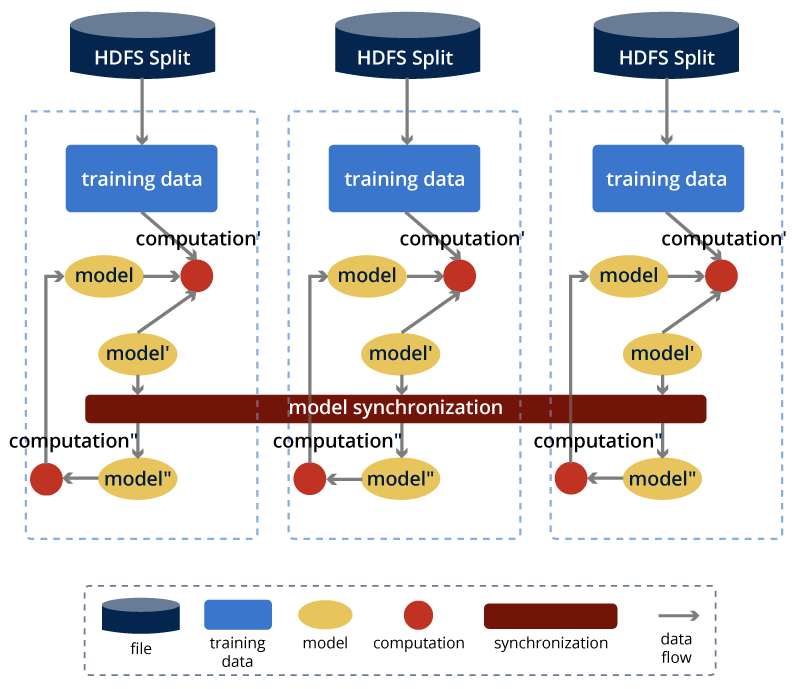This section has six tutorials demonstrating how to implement distributed machine learning algorithms with Harp framework.

Modern CPU and computation devices, such as many-core and GPU, provide powerful computing capacity but are usually challenging to deploy efficiently. To make it easier to design and implement distributed machine learning algorithms, we adopt a systematic process of parallelization with a focus on the computation models and their communication mechanisms. The tutorials provide examples of broadly used machine algorithms, including K-means, Multi-class Logistic Regression, Random Forest, Support Vector Machine, Latent Dirichlet Allocation and Neural Network, to represent the basics idea and steps for programming that port a non-trivial analysis algorithm from a sequential code into a distributed version.

These examples focus on the parallelization concept and expressiveness using Harp API, rather than performance optimization. In order to explore real applications, further tuning and advanced optimizations are necessary, which have been demonstrated in to the next section of real applications.

There are some general concepts that should be introduced before the tutorial starts:

• Input: Input data feed into the training process of the machine learning algorithm, which are also called ‘training data’, ‘data points’,‘examples’ or ‘instances’. Normally, input data are large and partitioned among the machines(nodes) in the cluster, calls ‘input splits’.

• Model: The “model” is the output generated when you train your “machine learning algorithm” with your training data-set. Here we focus on the data part. Training: Machine learning algorithms are normally iterative computation, processing the training data and update the model in each iteration and stop when reach the stop criterion.

• Prediction: Use the model learned from training data-set, and apply new data on it to get the outputs, the predictions.

• Data Parallelism: Training data are partitioned among nodes and all the splits are processed in parallel. It’s essential for big data problem.

• Model Parallelism: Model data are partitioned among nodes and model updates on each split are processed in parallel. It’s essential for big model problem.

The tutorial follows a similar structure, with 4 main sections:

## 1. Understanding Algorithm

This part gives simple background information on the target machine learning algorithm itself. The original algorithm does not need to have parallelism in consideration.

## 2. Parallel Design

This part illustrates the process of how to analysis the original sequential algorithm and to utilize the intrinsic parallelisms to design a parallel algorithm.

Under the Map-Collective programming model in Harp framework, there is a general pattern to do the parallel design for machine learning algorithms.##### Figure Map-Collective Programming Model on Iterative Machine Learning Algorithms

For training data, Harp will load the local data split on each node into memory in the initialization step of training, with no disk I/O to access the training data in the future. By default, the data split mechanism support by Hadoop Mapreduce are used.

For model data, Harp provides distributed dataset abstractions and collective communication and synchronization operations. Since the core computation of machine learning algorithms lies in the model update, the problems of model consistency and synchronization arise when parallelizing the core computation of model update. Harp has unique abstractions built upon collective synchronization mechanism, which is advantageous in expressiveness, efficiency and effectiveness for distributed machine learning applications.

The standard steps aim to answer four questions about the model design for a distributed machine learning algorithm based on its sequential algorithm.

• What is the model? What kind of data structure is applicable?

• What are the characteristics of the data dependency in model update computation – can they run concurrently?

• Which parallelism scheme is suitable – data parallelism or model parallelism?

• Which collective communication operation is optimal to synchronize the model?